## Table of ContentOpen Access

ARTICLE

# Hill Matrix and Radix-64 Bit Algorithm to Preserve Data Confidentiality

1 Department of Computer Science, National University of Technology, Islamabad, Pakistan
2 Department of Computing, Abasyn University, Islamabad, Pakistan
3 Department of Computer Science and Information Systems, College of Applied Sciences, AlMaarefa University, Riyadh, 13713, Saudi Arabia
4 Department of Computer Science, Faculty of Computer and Information Systems, Islamic university of Madinah, Medinah, 42351, Saudi Arabia
5 Department of Information Systems, Faculty of Computer and Information Sciences College, Majmaah University, 11952, Saudi Arabia
6 Department of Information Technology, College of Computer Sciences and Information Technology College, Majmaah University, Al-Majmaah 11952, Saudi Arabia
7 Department of Natural and Applied Sciences, Faculty of Community College, Majmaah University, Majmaah, 11952, Saudi Arabia

* Corresponding Author: Ali Arshad. Email:Computers, Materials & Continua 2023, 75(2), 3065-3089. https://doi.org/10.32604/cmc.2023.035695

## Abstract

There are many cloud data security techniques and algorithms available that can be used to detect attacks on cloud data, but these techniques and algorithms cannot be used to protect data from an attacker. Cloud cryptography is the best way to transmit data in a secure and reliable format. Various researchers have developed various mechanisms to transfer data securely, which can convert data from readable to unreadable, but these algorithms are not sufficient to provide complete data security. Each algorithm has some data security issues. If some effective data protection techniques are used, the attacker will not be able to decipher the encrypted data, and even if the attacker tries to tamper with the data, the attacker will not have access to the original data. In this paper, various data security techniques are developed, which can be used to protect the data from attackers completely. First, a customized American Standard Code for Information Interchange (ASCII) table is developed. The value of each Index is defined in a customized ASCII table. When an attacker tries to decrypt the data, the attacker always tries to apply the predefined ASCII table on the Ciphertext, which in a way, can be helpful for the attacker to decrypt the data. After that, a radix 64-bit encryption mechanism is used, with the help of which the number of cipher data is doubled from the original data. When the number of cipher values is double the original data, the attacker tries to decrypt each value. Instead of getting the original data, the attacker gets such data that has no relation to the original data. After that, a Hill Matrix algorithm is created, with the help of which a key is generated that is used in the exact plain text for which it is created, and this Key cannot be used in any other plain text. The boundaries of each Hill text work up to that text. The techniques used in this paper are compared with those used in various papers and discussed that how far the current algorithm is better than all other algorithms. Then, the Kasiski test is used to verify the validity of the proposed algorithm and found that, if the proposed algorithm is used for data encryption, so an attacker cannot break the proposed algorithm security using any technique or algorithm.

## Keywords

1  Introduction

Cloud cryptography is a secure data transmission system that can be used to protect data from attacks . Whenever an attacker tries to access the data, an attacker uses all possible phishing algorithms and techniques to gain access to the data from the cloud server . Data can be protected by two types of security , the first is external security and the second is internal security. External Security means protecting data from outside attacks, while the inner side means protecting data from inside attacks. A new technology called “mobile cloud computing” describes an infrastructure where data processing and storage occur outside mobile devices . The Internet of Things is a modern technology as well. The Internet of Things is a new technology expanding quickly in the telecom industry. Common outside cloud protection techniques include firewall, authentication, authorization, and intrusion detection and prevention systems , while inside cloud protection techniques include Cloudflare access control systems and Cloud Shell. Cloud servers are more prone to internal attacks, which are very difficult to detect. A Cloud Server cannot be saved unless its architecture is fully protected . By varying the traditional concurrency control algorithms in cloud-fog situations, it is possible to constantly avoid using the upstream communication channel from the clients to the cloud server .

An Intrusion Detection and Prevention System (IDPS) is a technique to detect and protect data against internal and external attacks on a cloud server . Whenever an attacker tries to attack a cloud server, different Intrusion Detection System techniques are used to detect the attack , which include a Host-Based Intrusion Detection System (SIDS) is used for it. In a Signature-Based Intrusion Detection System (SIDS), incoming packets are compared to existing packets . When the incoming packets match the existing packets, the user is considered a valid user . If the incoming packets do not match the existing packets, the user is considered an invalid user, and such users are detected with the help of SIDS . Network-Based Intrusion Detection System (NIDS) collects incoming and outgoing network traffic and prevents all harmful traffic . Whenever a user attempts to perform an unauthorized activity on a network that is not permitted, such a user can be detected through HIDS . An Anomaly-Based Intrusion Detection System (AIDS) can detect such users whenever an attacker tries to turn regular traffic into abnormal traffic . In cloud computing, techniques such as Intrusion Detection System (IDS), firewalls, and cloud flare can detect an attacker , and attackers can be blocked through these techniques, but data cannot be protected. If an attacker gains access to the cloud server through a phishing mechanism, it is challenging to protect the data from the attacker. The best way to protect cloud server data from attackers is to use cryptographic techniques . Different Acronyms or Notations are shown in Table 1.There are many data protection techniques, and algorithms available which if used correctly can detect all malware on cloud servers and keep data safe from attackers. Common data security techniques include data hiding, 3D image encryption, watermarking and information encryption . Data Hiding is an efficient technique to protect the data from attackers in which all internal information is hidden and only the information provided to the users which are required by the users. If this technique is used for data protection, all information can be prevented from unauthorized use and access to this data can be provided upon request to authorized users. Image encryption is the technique of encrypting confidential images using an encryption algorithm so that only authorized users may decipher them . A watermark is an identification code  that is indelibly incorporated into data and endures any decoding procedure.

Information Encryption is a technique that converts data into formats that are not easy to understand, known as non-readable formats. Cloud cryptography is divided into two types, the first is encryption and the second is decryption. Whenever a readable format is converted to a non-readable format, the process is called encryption . Encryption is a process in which data is secured using various steps and techniques  and converted into an unreadable format. Unreadable text is also called Ciphertext . Whenever cloud computing data is encrypted, the data can be encrypted in two ways. One is to use the same Key for encryption and decryption, while the other is to use two different keys for encryption and decryption . When data is encrypted and decrypted with the same Key, such process is called symmetric key cryptography. whenever data is encrypted and decrypted with two different keys, such process is called asymmetric key cryptography. Decryption is used whenever data is converted from non-readable to readable format .

Decryption is a technique that converts non-readable text to readable text . The steps and techniques used to decrypt the data must be the same as the encrypted time. Only authorized users can access this data if cryptography is implemented on cloud data . Encryption is always done on the sender side, while decryption is always done on the receiver side . When an attacker attempts to gain access to data through a snatching mechanism, the attacker cannot misuse that data despite receiving data from a cloud server  if the attacker interrupts the sender and receiver communication and tries to snatch the data, as shown in Fig.1. In this case, the attacker gets access to the data but cannot use it because an attacker can't decrypt it . When an authentic user tries to access the same data and uses a precise decryption mechanism, such a user will gain access to the data due to authenticity .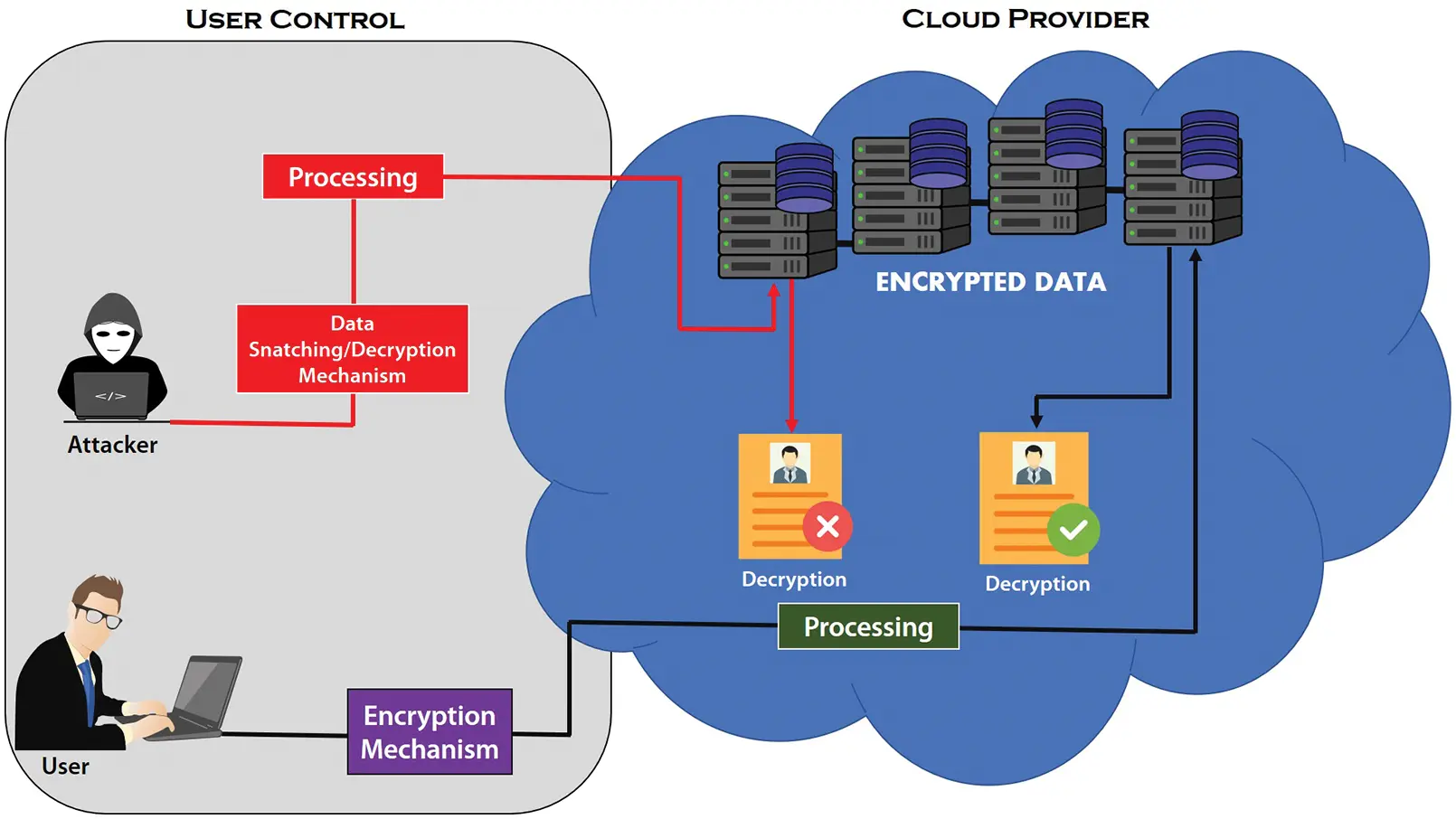Figure 1: Data accessibility through cryptography mechanism

1.1 Problem Formulation

Several Researchers have developed cryptographic algorithms to protect cloud data from being attacked and misused. Some researchers used predefined ASCII tables on matrix-based algorithms to encrypt data. With the help of a predefined ASCII table, the data can be taken in ciphertext format, but the data cannot be saved by using it. Some researchers have tried to secure data using one randomized Key for data encryption and decryption. Data can be encrypted and decrypted with the help of a key, but the data cannot be completely protected. The data can be more secure if encryption is done with one Key and decryption is done with two keys. Some researchers developed an algorithm by combining different algorithms and using a different key in each algorithm. If all these techniques are applied to any algorithm, the data can be encrypted but not wholly saved. Data can only be protected from an attacker if various techniques and methods are used for that data that is difficult for the attacker to access, as described in this article.

1.2 Contribution of Proposed Work

This article develops an efficient cryptography algorithm to secure data, protect cloud data from attack, and transmit data in a trusted environment. First, plain text is taken, and this Plaintext is encrypted with a static key. A custom ASCII table is generated to encrypt the data. Whenever an attacker tries to access data, the attacker always tries to use the default ASCII table. The attack rate can be reduced if the data is encrypted with a customized ASCII table instead of the predefined ASCII table. When the Plaintext and static Key are applied to the proposed algorithm, a dynamic key is generated at the time of encryption. A dynamic key operates on the text from which it is derived. When the Ciphertext is obtained, the ciphertext values are twice the original values. Whenever an attacker tries to decrypt data, the attacker tries to decrypt each character. But, when the number of encrypted texts is more than the original text, the data cannot be decrypted. Whenever a ciphertext is retrieved, certain values in that Ciphertext are repeated. When an attacker tries to apply a cryptanalysis mechanism or decrypt each character, the attacker will get confused in the Ciphertext because there will be too many repetitions of some values in the Ciphertext, But the data in the backend for each value will be different, which can only be obtained by dynamic Key, which is the novelty of the proposed algorithm.

The rest of the paper is organized as follows. Section 2 discusses previous work. Section 3 discusses the latest work methodology. The proposed methodology is evaluated in Section 4. Section 5 compares the previous work with the latest work. Section 6 discusses the conclusion.

2  Literature Review

Al-Shabi et al.  wanted to identify the performance of each cryptographic algorithm. To solve this paper, the Researcher collected different symmetric and asymmetric algorithms, compared all the algorithms used in these papers, and discussed the best algorithms for data protection and which algorithms can be used to secure data. According to the Researcher, if key-based algorithms for symmetric cryptography are developed that can be used to transmit cloud data in a secure form, such a mechanism will make it difficult for attackers to understand and decrypt data. After collecting the different techniques of each cryptographic algorithm, it was discussed that the efficiency and reliability of the symmetric cryptographic algorithms are higher than the asymmetric algorithm.

Nurhayati et al.  wanted to identify the time complexity of the Hill cipher algorithm, for which researchers introduced some techniques of the Hill cipher algorithm. In the Hill Cipher algorithm, the plain text was first equated with different numeric values, then the Key was generated, and the values were converted to matrix form. After that, the Key and numeric values were multiplied, and the Ciphertext was obtained. Then, the multiplicative results of the Hill cipher algorithm were applied to the Strassen algorithm to determine the time complexity of the Hill cipher algorithm.

Whenever data is transmitted over a network, attackers use a man-in-the-middle attack to taint the data. To solve this problem, Musa et al.  developed a Hill cipher algorithm, which put together different techniques that can be used to prevent such attacks. First, an algorithm was developed to protect the data from man-in-the-middle attacks and then to encrypt the data, and then a standard ASCII table was used to encrypt the data. Then, the ASCII values obtained from the Key and plain text were converted to binary values. The complement of each binary value was taken, and then these values were converted to decimals. After that, it was converted to ASCII values. The novelty of this paper is to use all the techniques that can be used to encrypt data in a secure environment.

Bentil et al.  wanted to develop a symmetric key encryption algorithm that could be used to transmit data in a reliable format across cloud networks. The Hill matrix algorithm was developed to encrypt the data and use the Key to solve this problem. The file was encrypted with a key from the sender; after that, the file was uploaded to the cloud server with the same Key. When the recipient decrypts the file, they access it using the same method and Key.

Rohim et al.  wanted to encrypt primary key data. Researchers modified the Hill Cipher algorithm to solve this problem and developed a new algorithm called the Hill Cipher Chain Algorithm. The purpose of developing this algorithm was to provide security to the Primary Key. In this algorithm, the primary Key was first converted to ASCII, then these ASCII values were converted to matrix form, and then a static key was generated. Then, the Ciphertext was obtained by multiplying the Key with the ASCII values, and the Ciphertext was stored in place of the primary Key.

Ahmed et al.  surveyed various papers, described many security techniques and algorithms, and provided as much information about cryptography as possible to researchers, students, and inexperienced people. The main purpose of this article is to identify the gaps in cryptography. The authors have identified two research gaps. The first gap is that researchers ignore the authentication process in security, whereas user authentication is the biggest problem for any organization. The second gap is that many researchers focus only on theory or partial implementation and ignore complete implementations. The authors have not discussed all the cryptographic algorithms in this paper but discuss the pros and cons of these algorithms, which can be used for new research.

Hossaim et al.  developed an advanced encryption algorithm to protect the data, in which three different algorithms were combined: play fair, caesar cipher and stream cipher to obtain a ciphertext. According to the researchers, if these effective algorithms are applied to cloud data, the attack rate can be reduced, and it will be difficult for an attacker to break the security of such an algorithm. To secure the data, the Ceaser cipher algorithm was first used, in which encryption was done using a randomized key, and a cipher text C1 was obtained. After that, the Playfair algorithm was implemented on C1 Ciphertext, and C1 ciphertext was obtained by encrypting the data, and then Ciphertext was obtained using the stream cipher algorithm. In these algorithms, data security is given by three different keys, and during decryption, these three keys must be used in sequence; otherwise, data decryption is impossible.

3  Proposed Algorithm

This paper developed an Advance Hill matrix algorithm and Radix-64-bit encryption to secure cloud data. Using it efficiently can protect the data from the attacker. If an attacker tries to decrypt the data, the attacker cannot decrypt it due to the use of different keys and the increase in the number of data

3.1 Customized ASCII Table

A customized ASCII table is a user-defined table that can be used only by the user who has developed the customized ASCII table. When an attacker develops a data decryption mechanism, the attacker always tries to use a predefined ASCII table. When data is converted from plain text to Ciphertext, various researchers use predefined ASCII tables that can be helpful for attackers in data decryption. If a customized ASCII table is used instead of the standard ASCII table, it will be difficult for an attacker to access the indexes of the customized ASCII table and store values in those indexes; as a result, the attacker will get data that has no relation to the original data instead of the original data. With the help of a customized ASCII table, the data can be provided with more security than the predefined ASCII table. If the customized ASCII table is used to secure the data, the algorithm's efficiency can increase further. A complete Customized ASCII table is shown in Table 2.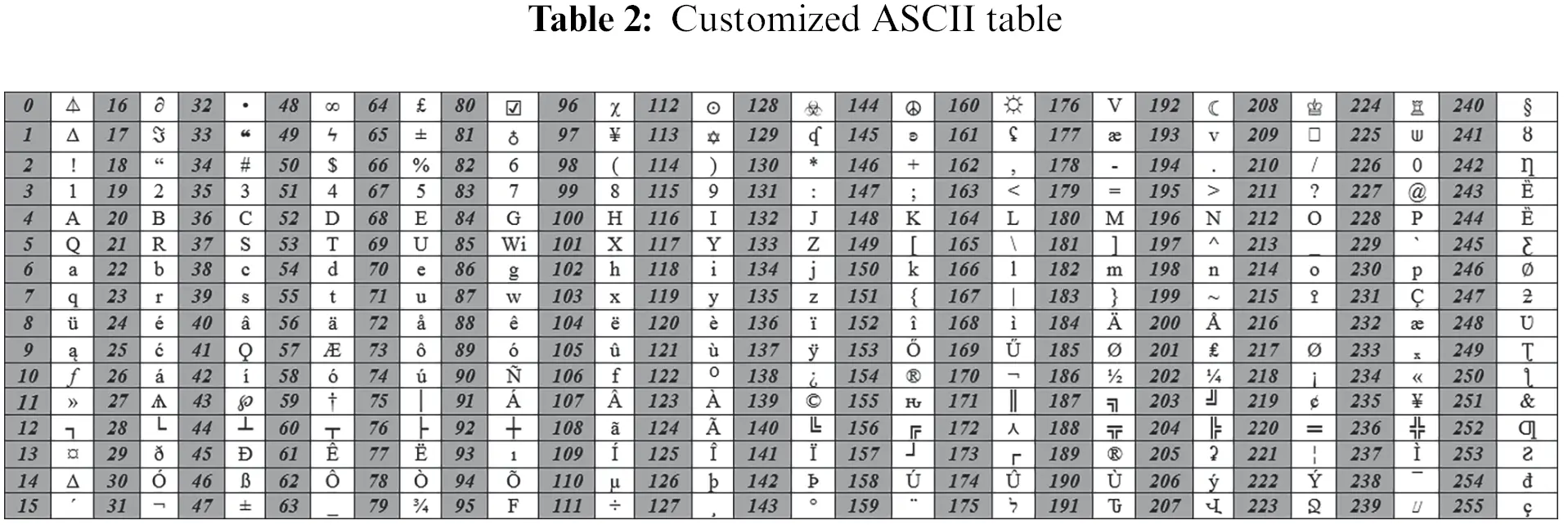3.2 Data Encryption Process

To prevent cloud data from being misused by the attacker, plain text is first to be taken, and this plain text is converted to its equivalent customized ASCII. Then these customized ASCII values are converted to binary. Afterward, the Key Generator generates a Randomized Key “K” in binary form; then, the Key is XORed with plain text binary values. After XORing the Key and plain text values, the Radix 64-bit encryption algorithm is implemented on the result obtained. In Radix-64-bit encryption, bits are divided into a 6-bits group and completed with the pairs. If the last pair is incomplete, the bits “0” are appended according to the length, and the decimal values are obtained. The radix 64-bit encryption mechanism makes the cipher values double the original values. When the value of the cipher text is higher than the original value, it will be difficult for an attacker to decrypt the cipher values. After getting the values from the radix 64-bit mechanism, the Hill Matrix algorithm is implemented. The Hill Matrix algorithm first converts decimal values to a 1 × 2 matrix. 1 × 2 matrix means one column and two rows. The formula “X = C–K” is used after converting to a 1 × 2 matrix. When XOR bits-results are converted to decimal, they are represented by “C.” When the Key generated by the key generator is converted to decimal, represented by “K.” When the “X = C – K” formula is implemented. A KeyJ” is generated. The value of “J” will be different for each text. After that, convert the values of “X” into the decimal format, and each ASCII value is equalized to its ASCII. Then cipher text is generated. A complete encryption process is shown in Fig. 2.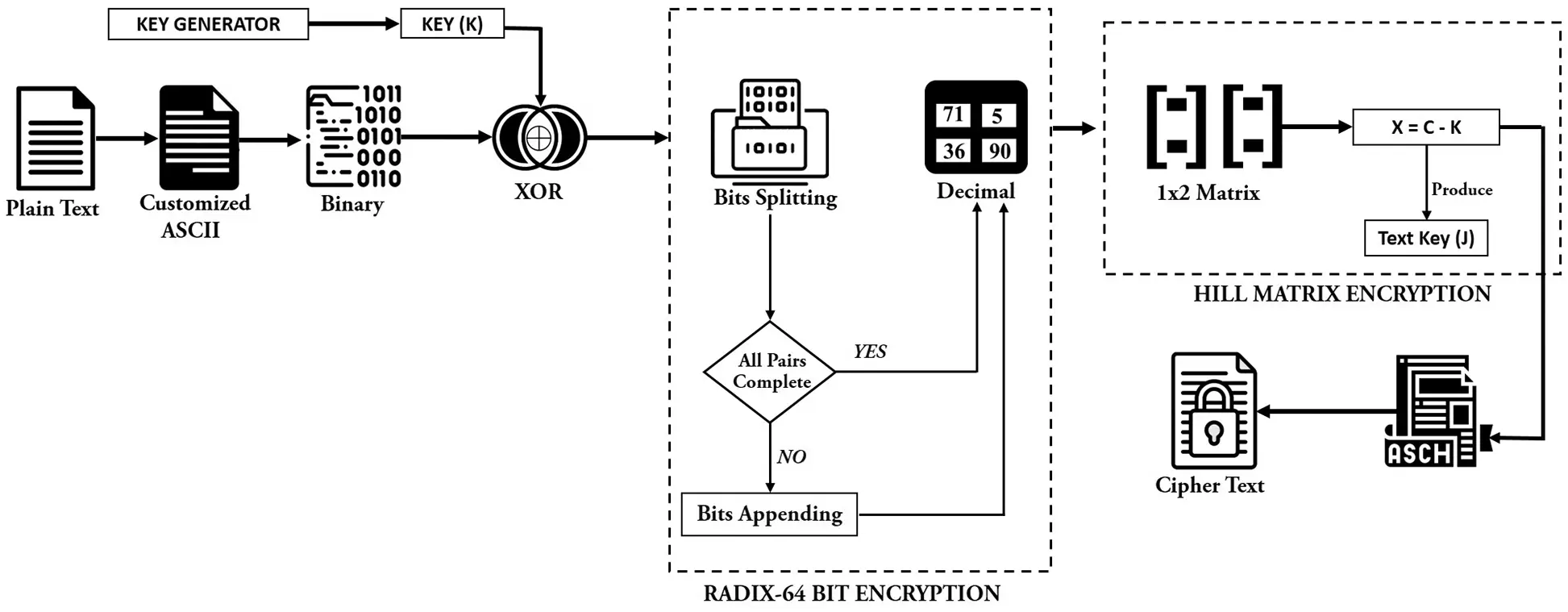Figure 2: Encryption mechanism

A Radix 64-bit encryption mechanism is developed to secure the data, increasing the amount of data. The reason for increasing the amount of data is that when an algorithm is implemented on every character, the attacker tries to change every character from non-readable text to readable text. If the number of original text increases during encryption, the attacker cannot get the original data when trying to decrypt each character, which is the best way to secure the data. In Radix 64-bit encryption mechanism, XOR results are first converted into a 6-bits binary to complete the pairs. If the pair is not complete, append a “0” to the end of the binary values, which are not complete, and each 6-bit value is converted to decimal format. If all 6-bit pairs are completed, then directly convert 6-bit values to decimal format; after that Hill matrix encryption algorithm is implemented on Radix 64 bits encryption results. Various steps to convert data from Plaintext to Ciphertext are shown in Fig. 3.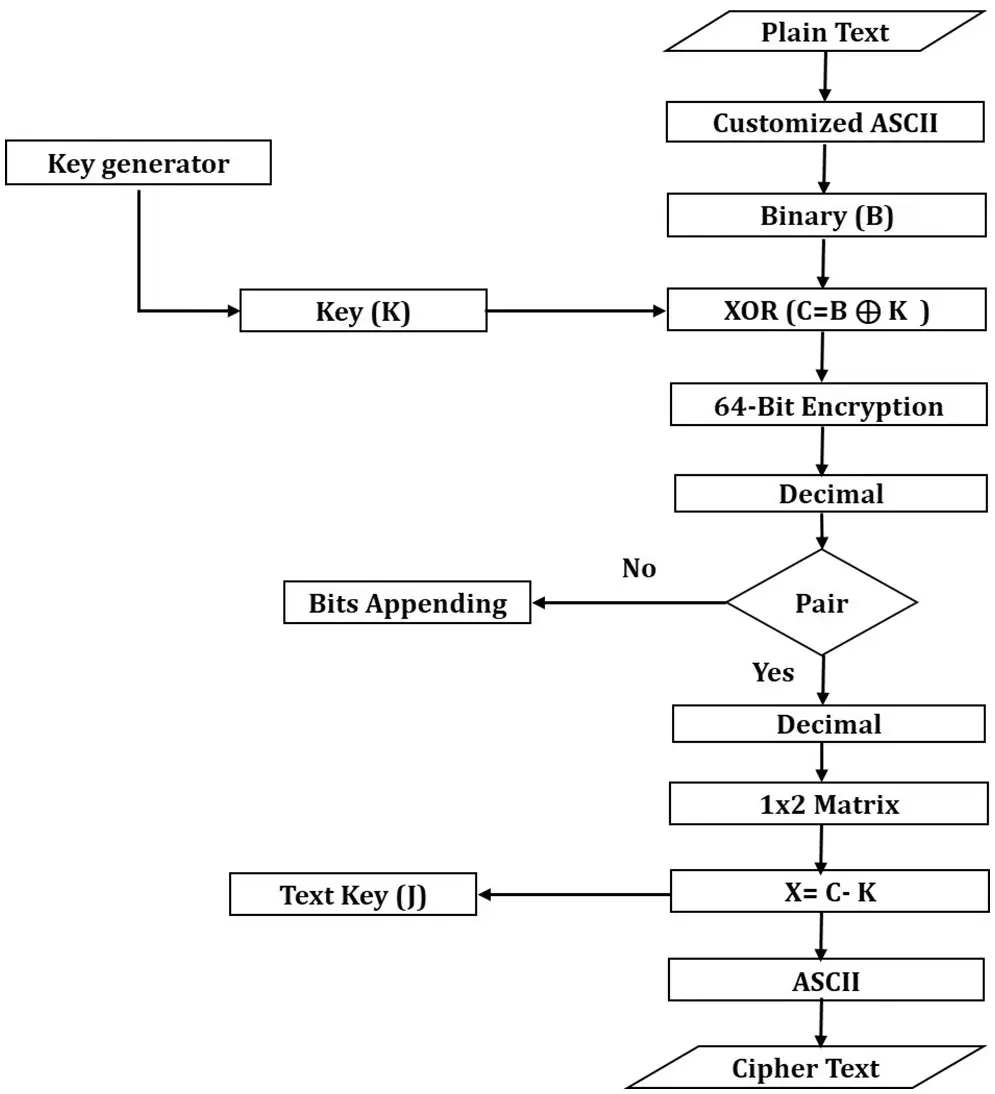Figure 3: Encryption flowchart

3.2.2 Hill Matrix Encryption

A Hill Matrix algorithm is developed to generate a unique key that belongs to this text to get the Key from plain text. The Key derived from each plain text is different from the other plain text, and this Key will only belong to that text. When the values are obtained from the Radix-64-bit encryption mechanism, the Hill matrix algorithm is implemented on these values. A 1 × 2 matrix algorithm is used in the Hill Matrix Algorithm. 1 × 2 matrix means one column and two rows in a matrix. In a 1 × 2 matrix, the Key “K” and XOR results “C” are converted to 1 × 2 matrix form, and then the formula “X = C–K” is implemented to obtain a random key for each text and a cipher value. After that, the values of “X” are converted into equivalent ASCII, and Ciphertext is obtained.

3.3 Data Decryption Process

To convert Ciphertext to plain text, the Ciphertext is converted to ASCII values, and then the ASCII is converted to a 1 × 2 matrix. After that, “C = A + J” is implemented, as shown in Fig. 3. When ASCII values are converted to a 1 × 2 matrix, the matrix values are represented by the “A,” while the “J” is the Key derived from the plain text. The results obtained from “A + J” is represented as “C,”. Various steps to convert the cipher text to plaintext are shown in Fig. 4.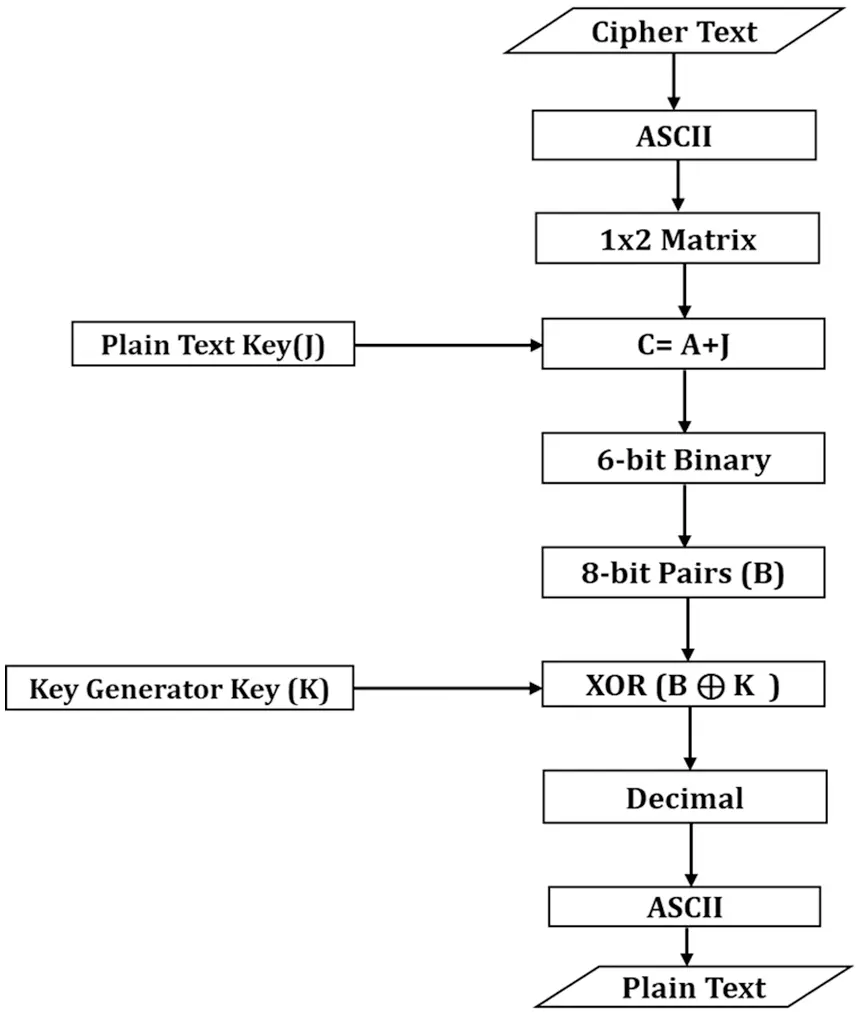Figure 4: Decryption flowchart

After that, each decimal value is converted to a 6-bits binary. After converting to bits “B”, these bits are XORed with Key “K”, and the result of XOR is converted to decimal. When the bits are converted to decimal, these decimal values are converted to equivalent ASCII, and then the plain text is obtained. The decryption Mechanism is shown in Fig. 5.Figure 5: Decryption mechanism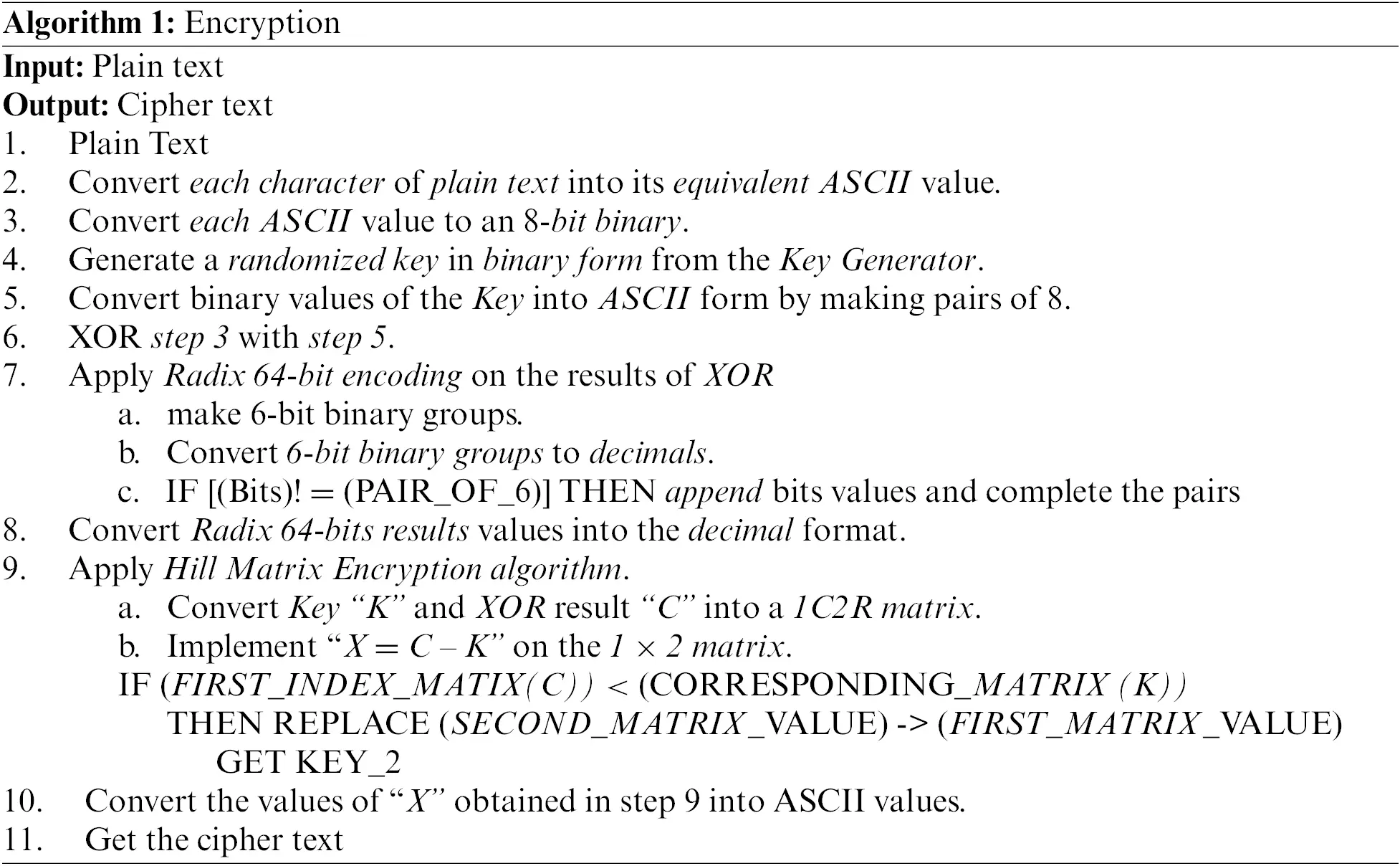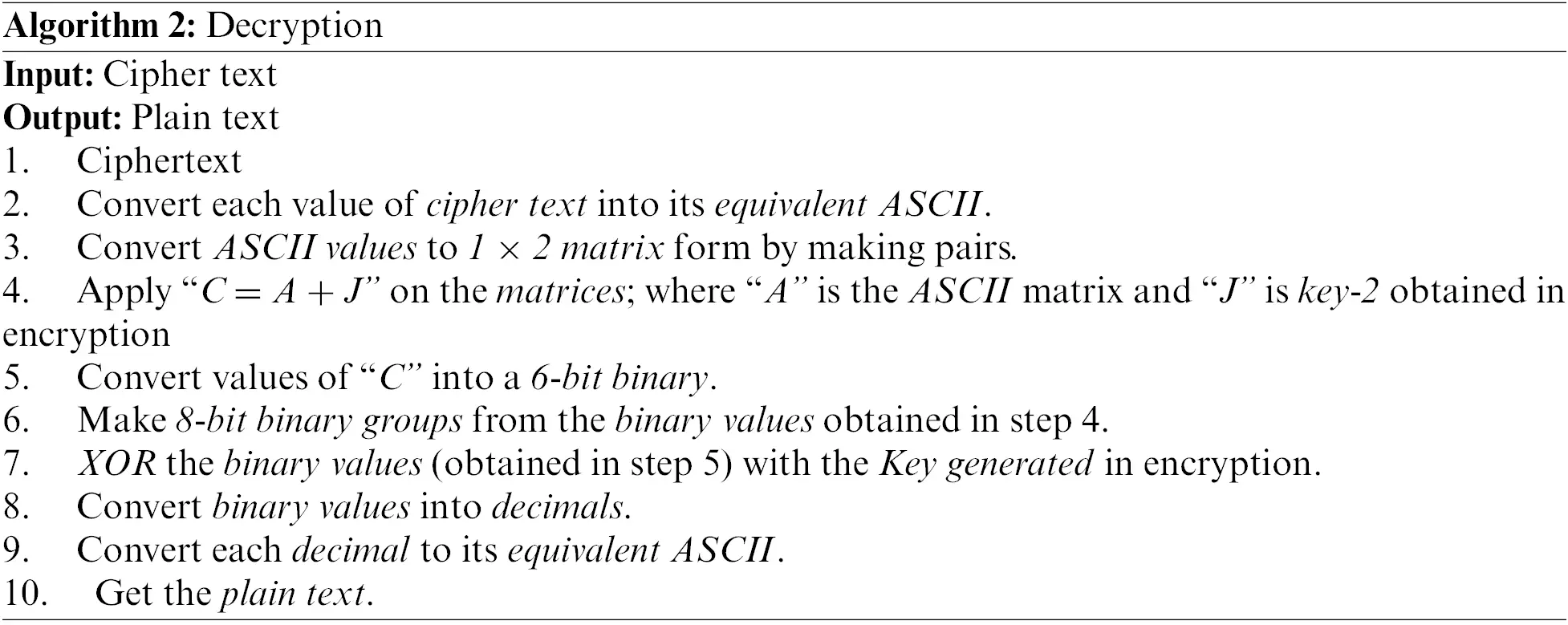4  Testing

4.1 Encryption Algorithm

Step 1: First, plain text is taken for encryption, as shown in Fig. 6.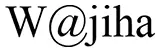Figure 6: Plain text

Step 2: Each plain text character is converted to its equivalent ASCII with the help of customized ASCII tables, as shown in Fig. 7.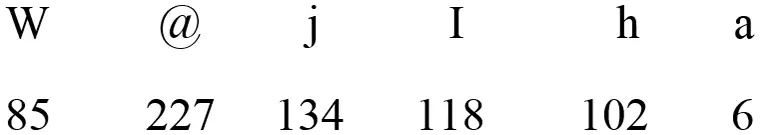Figure 7: Customized ASCII value of the plaintext

Step 3: After converting each character to ASCII, each value is converted to bits, as shown in Fig. 8.Figure 8: ASCII to binary conversion

Step 4: To provide data security, a Key “K” is generated from the Key Generator, and the binary bits “B” obtained from each text are XORed with the Key “K” according to the formula given below, as shown in Fig. 9.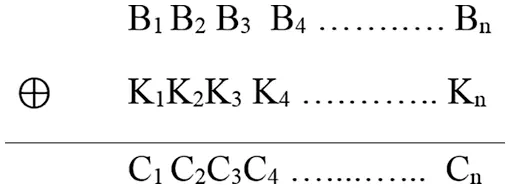Figure 9: XOR formula

After XORing the bits and Key, the result “C” is obtained, as shown in Fig. 10.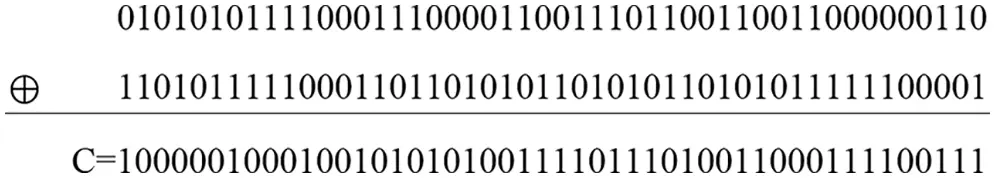Figure 10: XOR result of “B” and “K”

Step 6: To increase the number of cipher text, radix 64-bit encryption is implemented on XOR result, with the help of which the number of cipher text is increased from the actual data, for which groups of 6-bits are created. The groups are represented with “G”, as shown in Fig. 11. XOR results are represented with “C”, while the Key is represented with “K”, as shown in Fig. 9.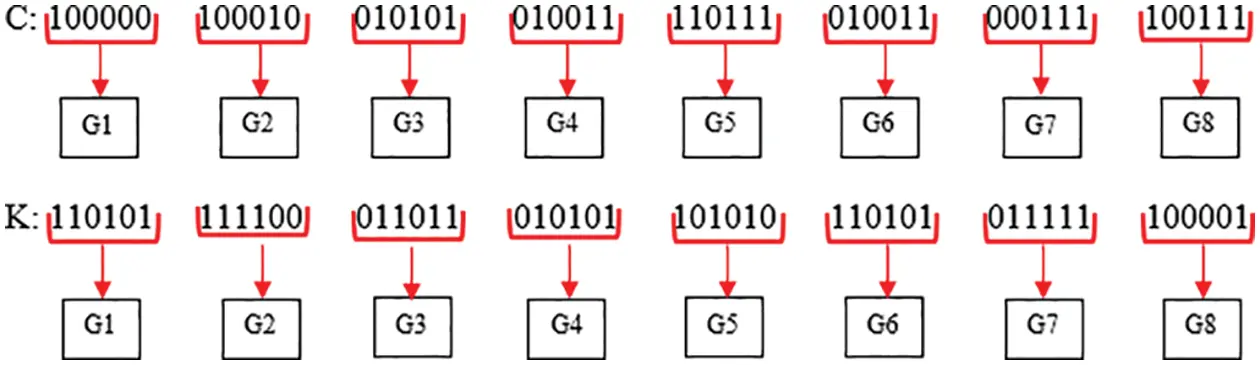Step 7: Binary values obtained from radix 64-bit encryptions are converted to decimal, as shown in Fig. 12.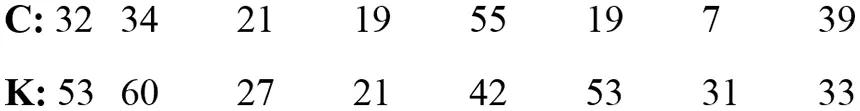Figure 12: Binary to decimal

Step 8: After converting to decimal, values are converted to matrix form by making pairs, as shown in Fig. 13.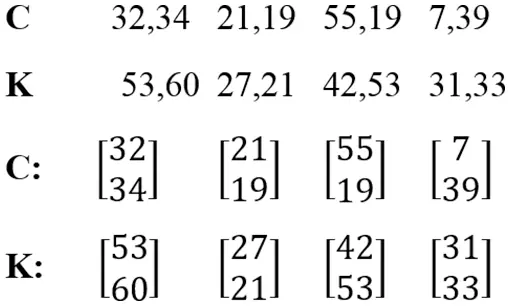Figure 13: 1C2R matrix

Step 9: After conversion to matrix form, “X = C–K” is implemented on the matrix, as shown in Fig. 14.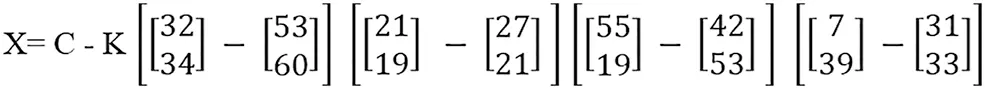Figure 14: Subtraction of matrix

If the value of any index of the first matrix is smaller than the second matrix, then the value of the second matrix is replaced by the value of the first matrix., as shown in Fig. 15.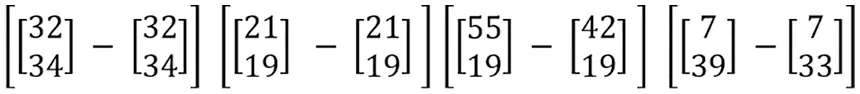Figure 15: Matrix index replacement

After replacing the “K” matrix value, obtained values are used as Key-2 in decryption. Values of Key-2 are represented with “J,” as shown in Fig. 16.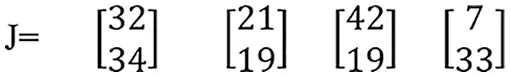Figure 16: Key2

After obtaining the value of “J,” results are obtained in matrix form using the formula “X = C–K,” as shown in Fig. 17.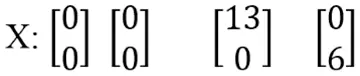Figure 17: Matrix subtraction result

Step 10: Each matrix value is converted to its equivalent ASCII, as shown in Fig. 18.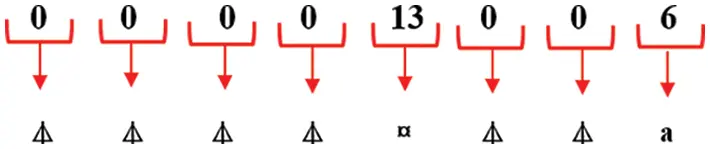Figure 18: ASCII values

Step 11: The result obtained after equalizing each character to its equivalent ASCII is cipher text, as shown in Fig. 19.Figure 19: Cipher text

4.2 Decryption Algorithm

Step 1: The cipher text, as shown in Fig. 20, is the input to the proposed decryption algorithm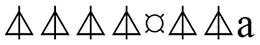Figure 20: Cipher text

Step 2: Each cipher text character is converted to its equivalent ASCII, as shown in Fig. 21.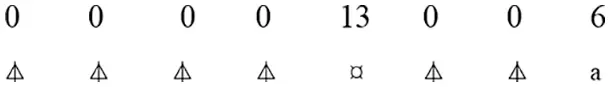Figure 21: ASCII values of cipher characters

Step 3: After converting to ASCII, values are converted to matrix form by making pairs, as shown in Fig. 22.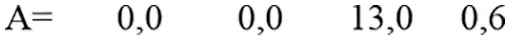Figure 22: Matrix form

Step 4: After converting to matrix form, values are added with the Key “J” derived from the text in encryption using “C = A + J,” as shown in Fig. 23.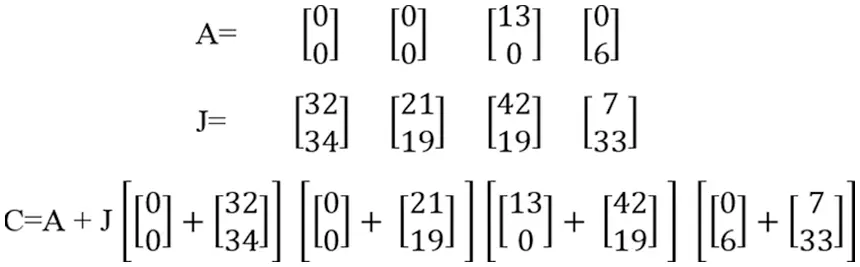After adding matrices, a result is obtained, as shown in Fig. 24.Figure 24: Result of matrix addition

Step 5: Decimal values obtained from matrix addition are converted to 6-bit binary, as shown in Fig. 25.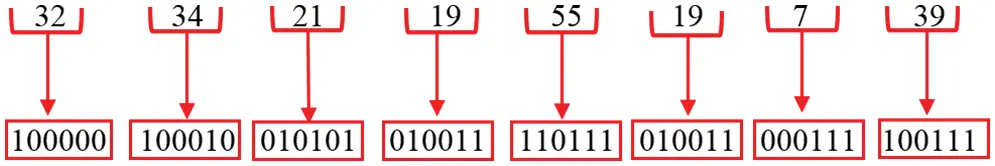Figure 25: 6-bit binary

6 Bit binary is converted to 8-bit binary to obtain plain text, as shown in Fig. 26.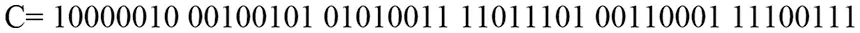Figure 26: 8-bit binary

Step 6: After obtaining 8-bit binary, binary bits are XORed with key-1 “K” generated in encryption using “Z = C ⊕ K,” as shown in Fig. 27.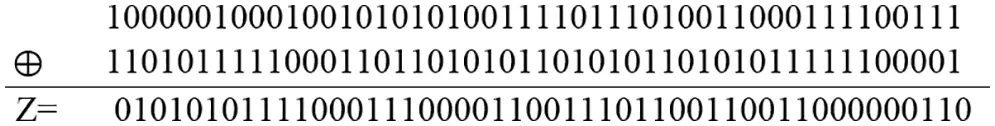Figure 27: XOR results

Step 7: The values obtained from XOR are converted to 8-bit binary pairs, and 8-bit binary pairs are converted to decimals, as shown in Fig. 28.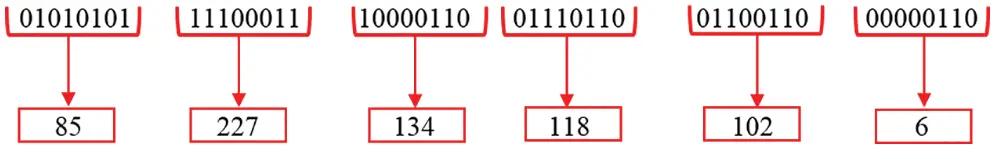Figure 28: Binary to decimal

Step 8: After decimal conversion, each decimal value is converted to its equivalent ASCII and plain text is obtained, as shown in Figs. 29 and 30.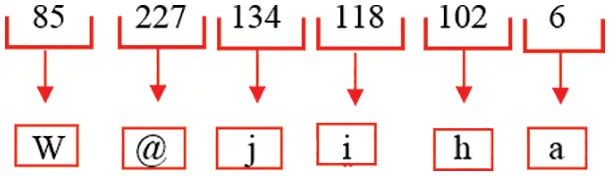Figure 29: ASCII values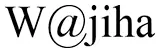Figure 30: Plain text

4.2.1 Testing-1

After encrypting and decrypting a simple plaintext, more different plaintexts are taken to check the algorithm's efficiency. Different keys are used, and the proposed algorithm is implemented to obtain different ciphertexts. Firstly, the Plaintext is taken, as shown in Fig. 31. This Plaintext is encrypted by a static key, “hello”.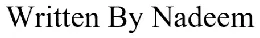Figure 31: Testing 1 plain text

After implementing the encryption mechanism on the Plaintext, an encrypted text is obtained, as shown in Fig. 32.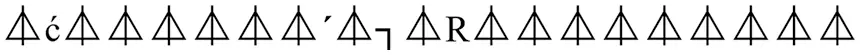Figure 32: Testing 1 ciphertext

4.2.2 Testing-2

A new plain text is taken to recheck the algorithm's efficiency, as shown in Fig. 33. A static key, “fish,” is used to encrypt the data, and an encryption mechanism is implemented on the plain text.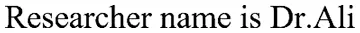Figure 33: Testing 2 plaintext

After implementing the encryption mechanism on the Plaintext, an encrypted text is obtained, as shown in Fig. 34.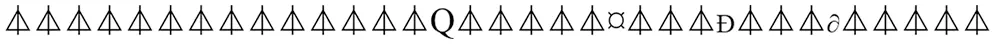Figure 34: Testing 2 ciphertext

4.2.3 Testing-3

The data is encrypted at different Key and text lengths, and different results are obtained. A complete sentence is taken as input to check the algorithm’s efficiency for the third time, as shown in Fig. 35, and this text is encrypted with a static key “cat”.Figure 35: Testing 3 plaintext

After implementing the encryption mechanism on the Plaintext, a ciphertext text is obtained, as shown in Fig. 36.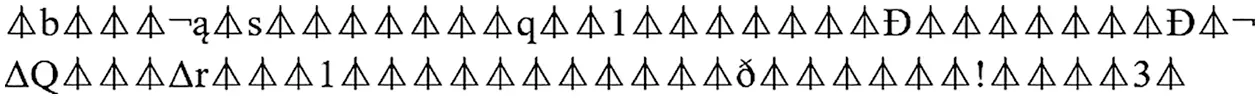Figure 36: Testing 3 ciphertext

In testing-1, the length of the plain text is 17, while the length of the Ciphertext is 22. In testing 2, the length of the plain text is 25, while the length of the Ciphertext is 34. In testing 3, the length of the plain text is 58, while the length of the Ciphertext is 74. As many plaintexts are converted into Ciphertext with the help of the proposed algorithm in which 95% of the Ciphertext consists of “⏃”.

In Table 3, whenever data is encrypted, each character is a corresponding cipher value. In very rare cases, it happens that 95% of the text in the Ciphertext is the same. When the cipher text is in such a form, it becomes very difficult for the attacker to develop a decryption mechanism, and it will be impossible to find the Key from such a ciphertext. The Ciphertext can be converted back to Plaintext by applying the same Key to the Ciphertext generated from the Plaintext. Data decryption is impossible unless the Key developed from the proposed paper is used during the decryption. 95% of the cipher values obtained from Testing-1 to Testing-3 are the same, which cannot be decrypted.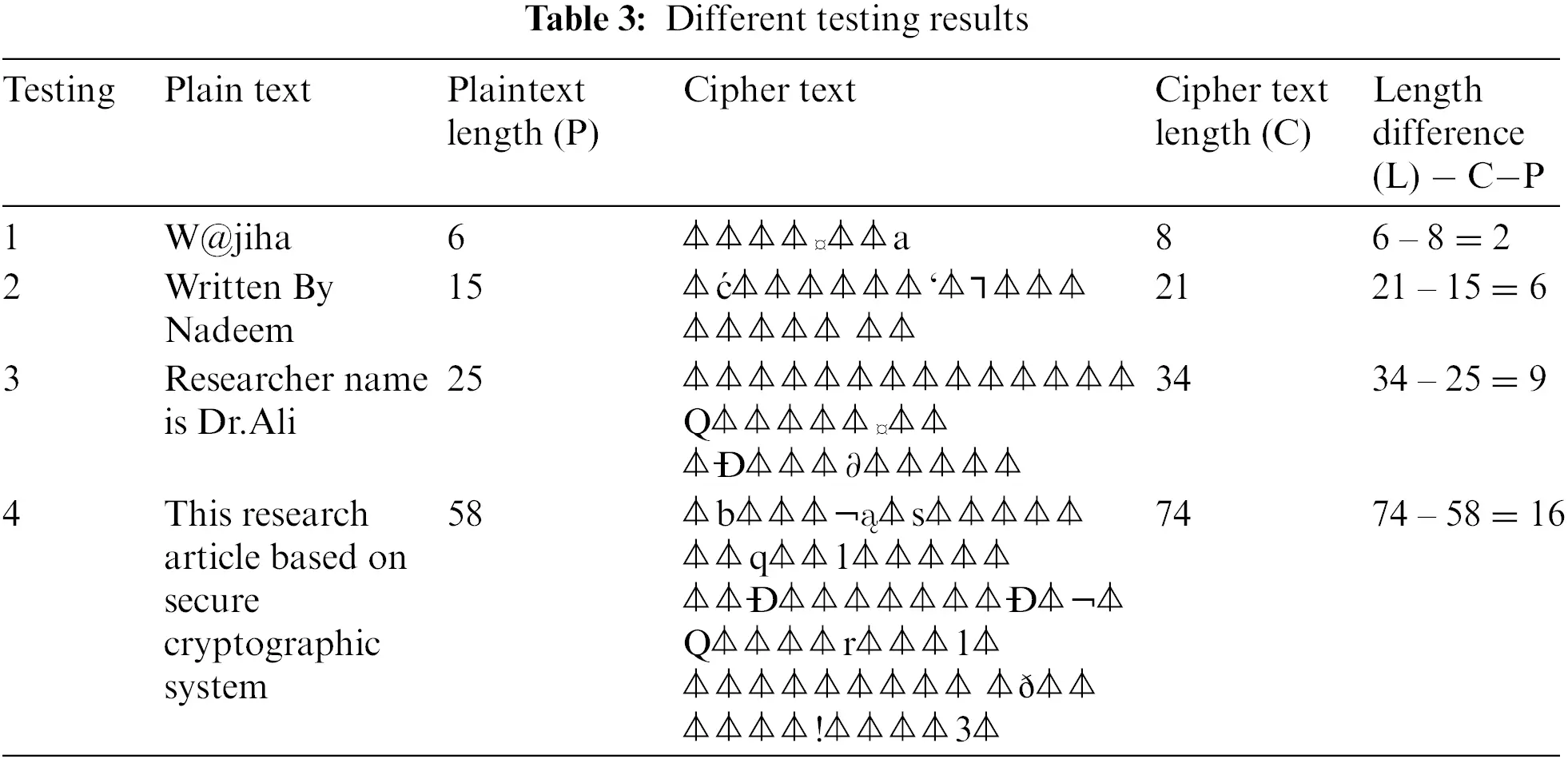After testing the algorithm at different times, the space and time complexity of encryption and decryption is determined, yielding different results, as shown in Tables 4 and 5.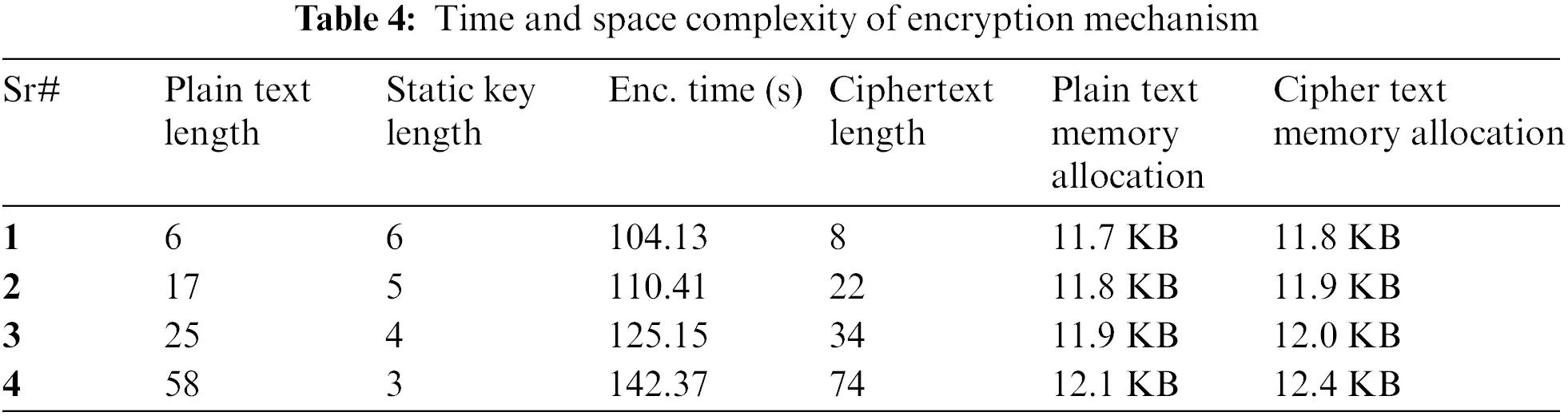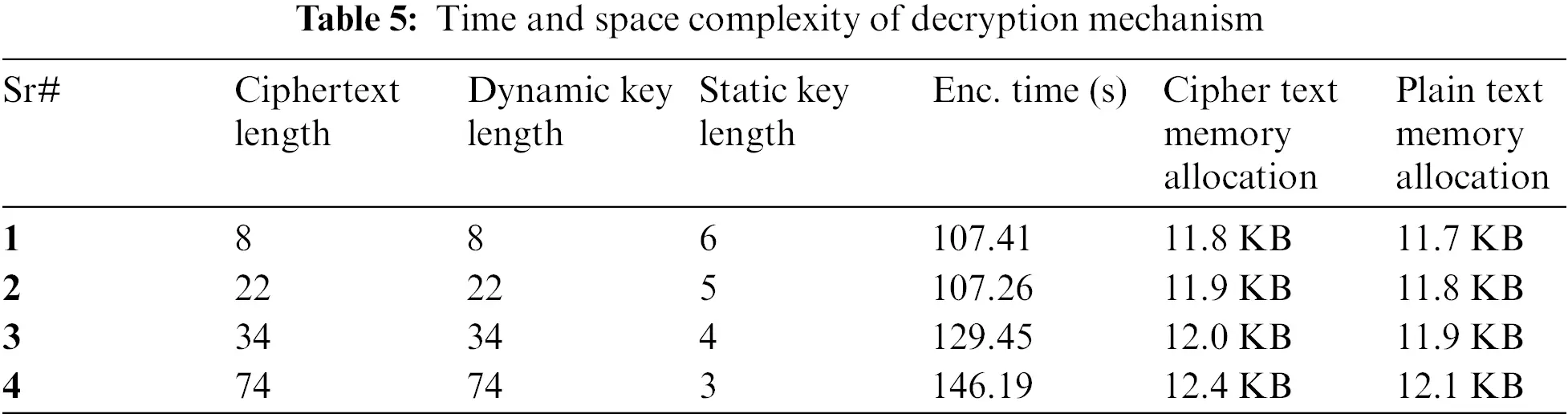4.3 Cryptanalysis

Cryptography is a data encryption technique in which data is converted into a format that cannot be easily deciphered. In cryptography, each character is encrypted with a Key to convert this data from readable to unreadable format. Whenever data is converted into a secret format or coded form, the process is called encryption. When a cryptographic code is deciphered, or the Key is obtained from that code, such a process is called cryptanalysis. In cryptanalysis, the Key is determined by using the Ciphertext. This paper uses two cryptanalysis algorithms to determine the proposed algorithm’s efficiency: The Key Cryptanalysis Algorithm by Plaintext and Ciphertext and the Key Cryptanalysis Algorithm by Ciphertext. In the proposed algorithm, data encryption is done with one Key, while decryption is done with two different keys. A static key is used for data encryption, while a random key is first used for decryption, and then a static key is used. A dynamic key is generated from each Plaintext and has access only to that Plaintext. Two different algorithms check the performance of the Key obtained from the proposed algorithm.

First, the efficiency of the dynamic Key obtained from the proposed algorithm is determined from the Key Cryptanalysis Algorithm by Plaintext and Ciphertext. Secondly, the efficiency of the Dynamic Key is determined by using the Key Cryptanalysis Algorithm by the Ciphertext. The reason for using two different algorithms is that the proposed algorithm's performance and each dynamic Key obtained from this algorithm can be determined in two different scenarios, as discussed in Sections 4.3.1 and 4.3.2.

4.3.1 Cryptanalysis by Plain Text and Cipher Text

In Key Cryptanalysis Algorithm by Plain text and Cipher text, an algorithm is applied to the Plaintext and Ciphertext to determine the Key “J”, as shown in Fig. 37. The reason for implementing the cryptanalysis on the dynamic Key is that if the proposed algorithm generates a unique key, then this Key can be obtained quickly or not. A cryptographic attack is always from an insider in cyberspace, and this attack can be possible when the attacker accesses cyberspace.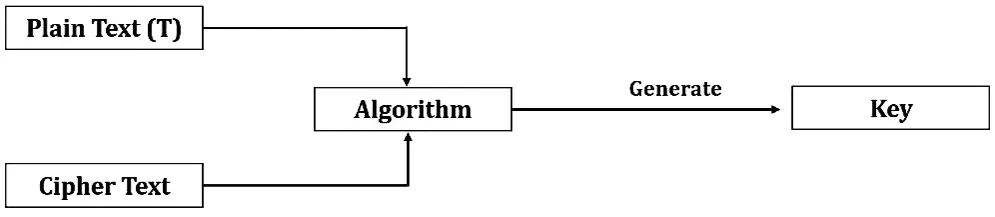Figure 37: Plain text and cipher text cryptanalysis

In the Key cryptanalysis algorithm by Plaintext and Ciphertext, firstly, a plain text “T” and one ciphertext “C” is taken and then identify the length of plain text “T” and Ciphertext “C”. Using any algorithm, the Key can be determined only when the length of the Plaintext and Ciphertext is equal. To check the proposed algorithm's novelty, testing is done using different plain text and keys and then obtaining the different cipher text length results, as shown in Table 6. After that, the length of different Ciphertext and Plaintexts is estimated. Any decryption algorithm can be applied only when both lengths are equal. If neither the Ciphertext nor the Plaintext is of equal length, one is made equal to the other, and then any algorithm can be implemented. In Table 6, the lengths of the two are not equal due to the different sizes of the Plaintext and Ciphertext. The length of the Plaintext cannot be equal to the Ciphertext, nor can it be equal to the Plaintext by adding values to the Ciphertext. This means that if the proposed algorithm is used and the attacker has Ciphertext and Plaintext, the attacker cannot know the dynamic Key, which is the novelty of the proposed algorithm.4.3.2 Cipher Text Cryptanalysis

Determining the Key “J” from the Ciphertext is very difficult, but with the help of the Index of coincidence, it may be possible to find the length of the Key. In this paper, to check the efficiency of the proposed algorithm, the ciphertext cryptanalysis algorithm is implemented on different ciphertext values, and the Key’s length is determined. The Ciphertext Cryptanalysis algorithm is shown in Fig. 38.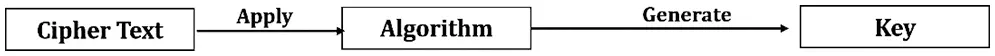Figure 38: Cipher text cryptanalysis

In ciphertext cryptanalysis, Plaintext is first taken, and the Ciphertext “C” is obtained by implementing the proposed algorithm on this plain text. After obtaining the Ciphertext, the length of the text is determined by implementing a cryptanalysis mechanism on the Ciphertext and then key identification is made.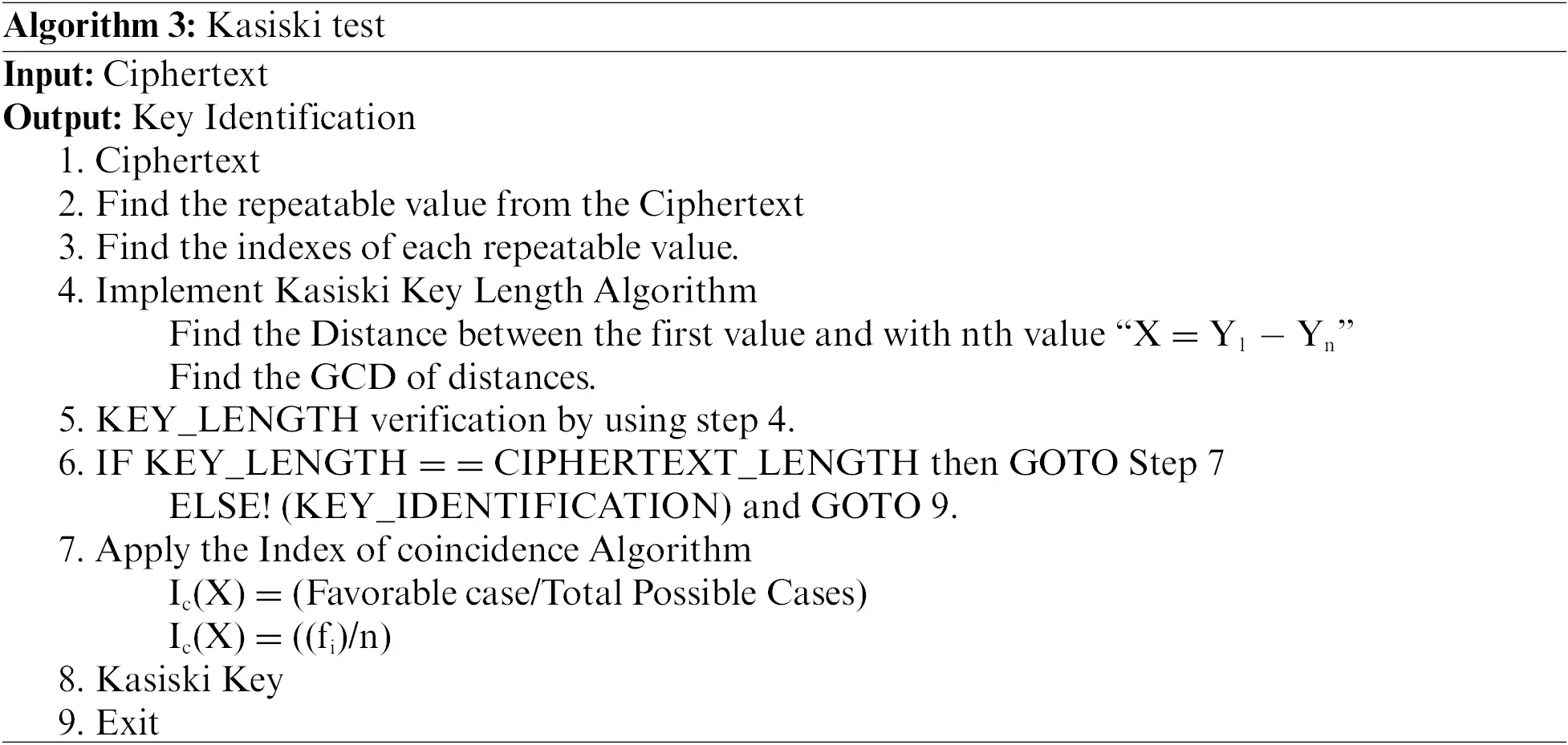The Kasiski Test is implemented on the ciphertext text to derive the Key. Firstly, find out the values that are repeated in the Ciphertext. If the values are repeating, there may be a possibility of finding the Key. If there is no repetition, the length of the Key used in the Ciphertext is equal to the length of the Plaintext. After finding the repeating values from the Ciphertext, the Index of each repeating value is determined. After finding out all the indexes, the Kasiski key length algorithm is implemented. In the Kasiski Key Length Algorithm, the Distance of index-1 is compared to all nth-indexes. After determining the Distance, the GCD of the values obtained from the Distance will be determined. The reason for finding the GCD is the probability of key length. For length verification, the dynamic Key obtained from the proposed paper is equalized to the Kasiski Key. If both are of equal length, Key identification is possible. In the Kasiski test, the key length is determined from the Ciphertext, but this paper compares the proposed algorithm key and the Key obtained by the Kasiski test to determine whether they are equal in length. If both lengths are equal, then the Index of coincidence algorithm cannot be implemented, as shown in Table 7. The Index of coincidence identifies the favorable cases and total possible cases. The favorable case means what the probability that two random numbers are equal. Total possible cases mean how many possible ways there are to find the length of the Key. In this paper, when Plaintext is converted to Ciphertext, a dynamic key is obtained during encryption, and 95% of the encrypted text is “⏃”. It is impossible to break such a text, nor can such a ciphertext be decrypted because the value “⏃” used in the Ciphertext will not allow any algorithm to execute, and this text can be decrypted only by the Dynamic Key developed by this proposed algorithm.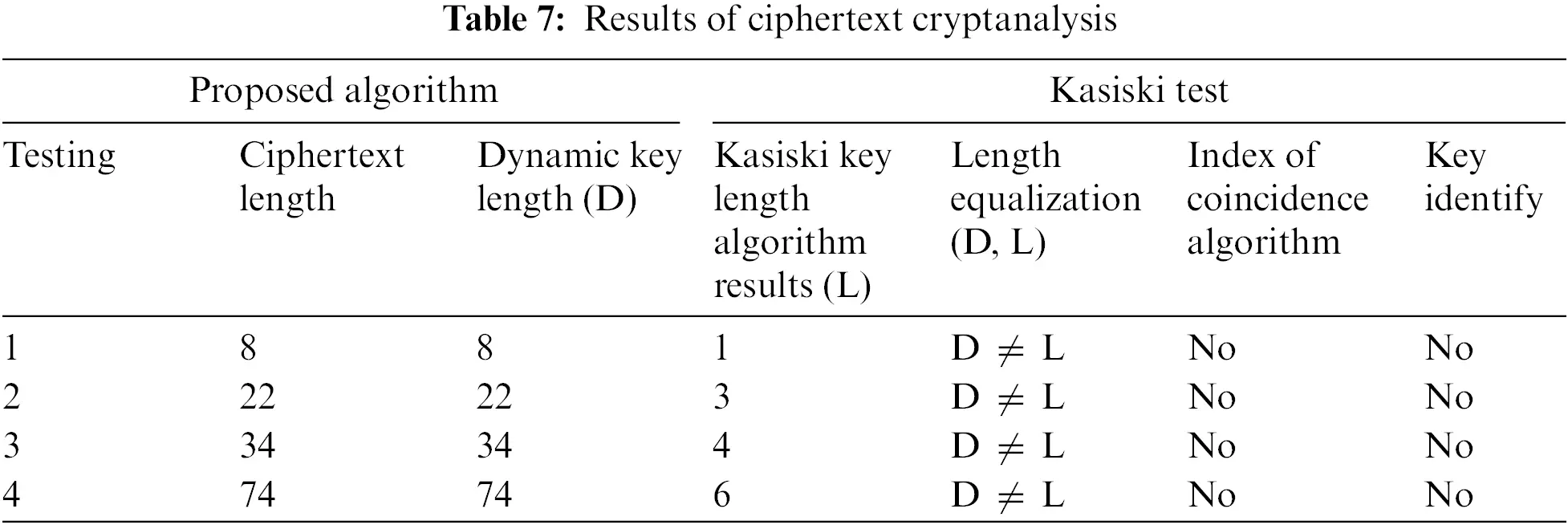After implementing the cryptanalysis algorithm in two different scenarios, it is concluded that 95% of the Ciphertext obtained from the proposed algorithm depends on one character that is impossible to break. The attacker’s first attempt is to implement a Kasiski test whenever an attacker tries to implement any mechanism. The probability of finding the actual Key from the Ciphertext can be done with the help of the Kasiski test. In this paper, key identification is made in two different scenarios, but no mechanism can be implemented by repeating a character. When an attacker obtains such encrypted data, the attacker will be confused with the Ciphertext, and no mechanism will be able to enforce that Ciphertext. If the proposed algorithm is used for cloud data, its first advantage is to obtain a dynamic key, while another advantage is to obtain the text that needs to be decrypted with the same Key that was generated in the encryption. The decryption of the text is not possible until the dynamic Key is used, and any mechanism cannot obtain the dynamic Key.

5  Comparative Analysis

Different researchers have developed different cryptographic algorithms to secure cloud data. In 2019 , the researchers surveyed different papers and compared all the symmetric and asymmetric algorithms used in these articles and discussed that the reliability and performance of the symmetric algorithm are higher than that of the asymmetric algorithm. Instead of comparing different algorithms, the attack rate can be reduced if all the best techniques are combined and an efficient algorithm is developed, but the researchers only compared all algorithms, which is the flaw of this paper.

In 2020 , the Researcher proposed a Hill cipher algorithm in which encryption was done by implementing the multiplication of Key numeric values with plain text numeric values using a standard ASCII table. After that, the results of the Hill Cipher algorithm were implemented on the Strassen algorithm to identify the time complexity. The drawback of this paper is that this Hill Cipher algorithm is unreliable for broadcasting data over the network because such algorithms can be easily decrypted.

In 2021 , researchers collected various techniques from various papers and developed a Hill cipher algorithm that includes a standard ASCII table, static Key, binary conversion, and complement of each binary. This paper does not develop state-of-the-art techniques but uses techniques already used to generate ciphertexts. Instead of using already existing techniques, the algorithm’s functionality can be enhanced if the latest techniques are used in the algorithm, which is the drawback of this paper.

In 2021 , researchers developed a symmetric hill cipher encryption algorithm that encrypts and decrypts data with the same Key. After encrypting the data, the data and the Key are uploaded to the server. Instead of uploading the Key to the cloud server, an algorithm was developed in which a key was obtained from each plain text, and one Key was uploaded to the cloud server, and the other users had it. This paper’s drawback is that such an algorithm can reduce the cloud attack rate.

In 2022 , the Researcher modified the Hill cipher algorithm and introduced a newer algorithm called the Hill cipher chain algorithm, which encrypted the primary text key. This algorithm was developed to encrypt the primary Key only. Instead of encrypting only the primary Key, if an efficient algorithm such developed that can encrypt every text data, then the algorithm’s reliability could be increased, which is the drawback of this paper. A complete Comparative analysis of previous and latest work is shown in Table 8.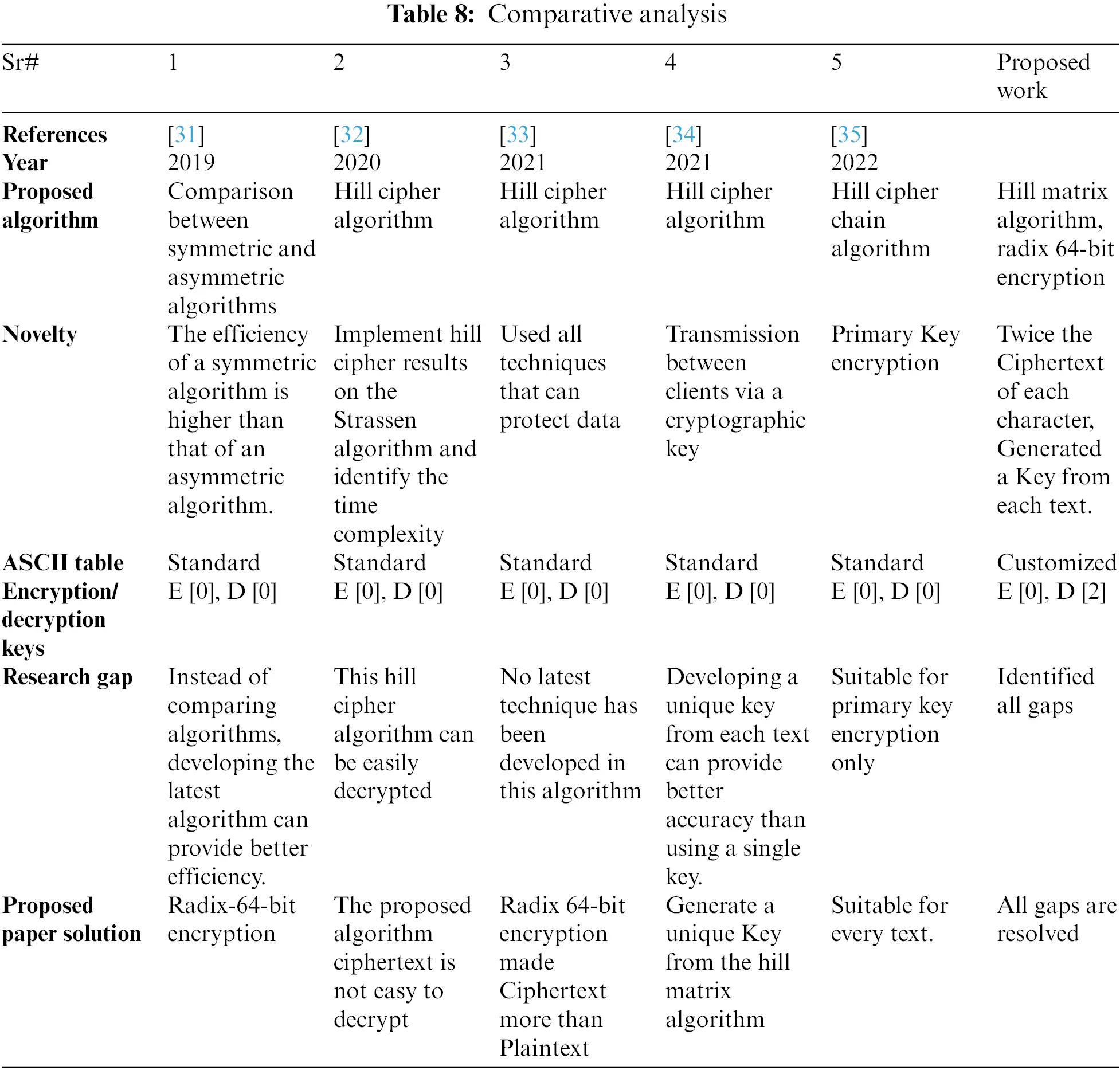Novelty of Proposed Work

Some researchers compared different techniques of different papers. Some researchers have encrypted files with a key and uploaded the files to a cloud server. Some researchers have encrypted data using the encryption Hill matrix formula. These algorithms can be used to encrypt data but cannot provide secure and reliable protection between clients. If these algorithms are provided with additional security or encrypted with a key that can only be used in the text from which it is created, it will make it impossible for an attacker to obtain the Key of each text in the data. This will make it difficult to decrypt the data. The advantage of deriving the Key from each encryption time text is that the data can be transmitted across the network in a reliable form. Various researchers  developed different algorithms to secure data in which the length of the plaintext and the length of the ciphertext was same. A static key was then used to secure the data, with the help of which the data was encrypted and decrypted. In very few cases it happened that the values were the same in ciphertexts obtained by different algorithms. It is very easy to implement a cryptanalysis algorithm on such a ciphertext. When 95% of the ciphertext will be same and the resulting of ciphertext will dependent on the derived key. The attacker will not be able to obtain the data, until the attacker has the key that linked to the ciphertext, which is the novelty of paper. In this paper, an efficient cryptography algorithm is developed for securing the cloud data, reducing the number of attacks if used on a cloud server.

There are many advantages to using this algorithm to provide security to cloud data. First, a customized ASCII table is developed. The reason for using a customized ASCII table instead of a standard ASCII table is to prevent data from attackers and decryption. Whenever an attacker converts Ciphertext to Plaintext, the attacker consistently implements the standard ASCII table on the Ciphertext. If a customized ASCII table is used instead of the standard ASCII table, it is difficult for the attacker to get the index number of the cipher values. Second, a Key is obtained from a Key Generator. Then, the Key is XORed with values obtained from a customized ASCII table to make data decryption impossible for an attacker. Third, a Radix-64-bit encryption mechanism that implements the technique of splitting bits is developed. The most significant advantage of using radix-64-bit encryption is that the number of cipher text is double that of the original text. When an attacker tries to decrypt the cipher data, the attacker tries to decrypt each cipher value, but due to the Radix-64-bit algorithm, the attacker will not be able to get the original data even if he uses a customized or standard ASCII table. Fourthly, the Hill matrix algorithm is implemented on the Radix-64-bit algorithm. A key is obtained from Plaintext with the help of the Hill matrix algorithm. The Key derived from each plain text will work only up to that text. A plain text key will not work in other plain text, and each Key can be used only in the exact Plaintext from which it is derived. When this algorithm is used for data transmission, 95% of the cipher text values will depend on “⏃”, making it difficult for an attacker to derive the actual values from the cipher values “⏃” and when the same values are obtained repeatedly in the cipher text, it will be impossible for an attacker to get the original data which is the novelty of this paper. If this algorithm is used for data transmission, it will be impossible for an attacker to break the security of each step, and it won’t be easy to obtain the Key from each text to decrypt the data.

6  Conclusion

After securing the cloud data and making comparisons with different papers, it is concluded that cloud cryptography is a technique that can be used to protect data from attackers. If an attacker gains access to the cloud server, it is necessary to decrypt that data to use the cloud data. Cloud data can only be secure if the algorithm built for it is efficient and reliable. Whenever an attacker attacks cloud data, there are two possible reasons. The first reason is using algorithms that can encrypt data, but these algorithms do not have complete security mechanisms and can cause attacks on data. The second reason is to decrypt the cipher text through the ASCII table. Whenever an attacker tries to decrypt the cloud data, the attacker’s first attempt is to use the ASCII table, which can be helpful for the attacker.

In this paper, an algorithm has been developed to secure cloud data from which 95% of the text obtained contains a symbol that cannot be easily understood or broken. To decrypt the data obtained from the proposed algorithm, it is necessary to use the same key which has been used to encrypt the data. The radix 64-bit algorithm is used to double the number of encrypted data from the number of original data. Whenever an attacker tries to decrypt the data, the attacker tries to decrypt each text data but this data has no relation with the original data. Data is secured in different phases. If an attacker develops a mechanism to decrypt the data, the attacker will not be able to break the security of the data due to the presence of security mechanisms in each phase. If the algorithm developed in this article is used for a real-time environment, it will be difficult for attackers to crack and understand such techniques, providing a reliable environment for data transmission. If this algorithm is used to secure the data and some more advanced features are added, every decryption step will be impossible for the attacker.

In the future, the existing algorithm will be modified by adding some latest techniques to secure the data further and broadcast it over the network. When an attacker tries to decrypt the data, the data will be decrypted by the receiver public key, plain text generated Key and a static key, and the number of cipher text will be tripled from the original number, which will be difficult for the attacker to understand how many characters the original data contains. After that, the existing algorithm will compare with the algorithm, and the performance of each algorithm will be measured. Then there will be a discussion about which algorithm can provide better data transmission.

Acknowledgement: The authors deeply acknowledge the Researchers supporting program (TUMA-Project-2021-27) Almaarefa University, Riyadh, Saudi Arabia for supporting steps of this work.

Funding Statement: This research was supported by the Researchers supporting program (TUMA-Project-2021-27) Almaarefa University, Riyadh, Saudi Arabia.

Author Contributions: A. A. Conceptualization; M. N Software, Writing—review & editing; S. R Review & editing; S. W. Z Methodology; A. K. D Editing; Z. A Review; R. A Editing; S. A. Review and Funding acquisition.

Conflicts of Interest: The authors declare that they have no conflicts of interest to report regarding the present study.

## References

1. M. Nadeem, A. Arshad, S. Riaz, S. S. Band and A. Mosavi, “Intercept the cloud network from brute force and ddos attacks via intrusion detection and prevention system,” IEEE Access, vol. 9, pp. 152300–152309, 202
2. S. Ustebay, Z. Turgut and M. A. Aydin, “Intrusion detection system with recursive feature elimination by using random forest and deep learning classifier,” in 2018 Int. Congress on Big Data, Deep Learning and Fighting Cyber Terrorism (IBIGDELFT), Ankara, Turkey, pp. 71–76, 2018.
3. S. K. Wagh, V. Pachghare and S. Kolhe, “Survey on intrusion detection system using machine learning techniques,” International Journal of Computer Applications, vol. 78, no. 16, pp. 30–37, 201
4. C. Stergiou, K. E. Psannis, B. GyuKim and B. Gupta, “Secure integration of iot and cloud computing,” Future Generation Computer Systems, vol. 78, no. 54, pp. 964–975, 2018.
5. N. G. McDonald, “Past, present and future methods of cryptography and data encryption,” in A Research Review. University of Utah, 2020.
6. M. M. Islam, M. Z. Hasan and R. A. Shaon, “A novel approach for client side encryption in cloud computing,” in 2019 Int. Conf. on Electrical, Computer and Communication Engineering (ECCE), Cox’sBazar, Bangladesh, pp. 1–6, 2019.
7. A. Al-Qerem, M. Alauthman, A. Almomani and B. B. Gupta, “Iot transaction processing through cooperative concurrency control on fog-cloud computing environment,” Soft Computing, vol. 24, no. 8, pp. 5695–5711, 2020.
8. G. Luo, “Research on computer network security problems and protective measures under the background of big data,” Journal of Physics: Conference Series, vol. 1607, no. 1, pp. 1–6, 2020.
9. B. Ma and Y. Q. Shi, “A reversible data hiding scheme based on code division multiplexing,” IEEE Transactions on Information Forensics and Security, vol. 11, no. 9, pp. 1914–1927, 2016.
10. I. Meraouche, S. Dutta, H. Tan and K. Sakurai, “Neural networks-based cryptography: A survey,” IEEE Access, vol. 9, pp. 124727–124740, 2021.
11. T. Sommestad, H. Holm and D. Steinvall, “Variables influencing the effectiveness of signature-based network intrusion detection systems,” Information Security Journal: A Global Perspective, vol. 31, no. 6, pp. 711–728, 2021.
12. S. Kumar, S. Gupta and S. Arora, “Research trends in network-based intrusion detection systems: A review,” IEEE Access, vol. 9, pp. 157761–157779, 2021.
13. K. Letou, D. Devi and J. Y. Singh, “Host-based intrusion detection and prevention system (HIDPS),” International Journal of Computer Applications, vol. 69, no. 26, pp. 28–33, 20
14. V. E. Jyothi, B. D. C. N. Prasad and R. K. Mojjada, “Analysis of cryptography encryption for network security,” IOP Conference Series: Materials Science and Engineering, vol. 981, no. 2, pp. 1–7, 2020.
15. K. B. Gyam and G. O. Bempah, “Comparison of a proposed algorithm and hill cipher algorithm in cryptography,” The International Journal of Scientific and Research Publications (IJSRP), vol. 10, no. 12, pp. 355–365, 2020.
16. Y. Sharma, H. Gupta and S. K. Khatri, “A security model for the enhancement of data privacy in cloud computing,” in 2019 Amity Int. Conf. on Artificial Intelligence (AICAI), Dubai, United Arab Emirates, pp. 898–902, 2019.
17. T. Alawiyah, A. B. Hikmah, W. Wiguna, M. Kusmira, H. Sutisna et al., “Generation of rectangular matrix key for hill cipher algorithm using playfair cipher,” Journal of Physics: Conference Series, vol. 1641, pp. 1–5, Voronezh, Russia,2020.
18. X. Wang, X. Wang, B. Ma, Q. Li and Y. -Q. Shi, “High precision error prediction algorithm based on ridge regression predictor for reversible data hiding,” IEEE Signal Processing Letters, vol. 28, pp. 1125–1129, 2021.
19. R. Han, “A hash-based fast image encryption algorithm,” Wireless Communications and Mobile Computing, vol. 2022, no. 6, pp. 1–8, 2022.
20. A. Iacovazzi and Y. Elovici, “Network flow watermarking: A survey,” IEEE Communications Surveys & Tutorials, vol. 19, no. 1, pp. 512–530, 2017.
21. B. Swathi, S. D. Bhaludra and S. Raveendranadh, “Secure file storage in cloud computing using hybrid cryptography algorithm,” International Journal of Advance Research in Science and Engineering, vol. 6, no. 11, pp. 334–340, 2017.
22. D. Dahanukar and D. Shelke, “A review paper on cryptography and network security,” International Journal of Advanced Research in Science, Communication and Technology, vol. 12, pp. 108–114, 2021.
23. Q. Li, X. Wang, B. Ma, X. Wang, C. Wang et al., “Concealed attack for robust watermarking based on generative model and perceptual loss,” IEEE Transactions on Circuits and Systems for Video Technology, vol. 32, no. 8, pp. 5695–5706, 2022.
24. M. T. Bemila, K. Kundar, L. Jain, S. Sharma and N. Makasare, “Comparative study of various security algorithms applicable in multi-cloud environment,” Int. J. Advanced Research in Computer and Communication Engineering, vol. 5, no. 3, pp. 460–463, 2016.
25. S. Gao, R. Wu, X. Wang, J. Wang, Q. Li et al., “A 3D model encryption scheme based on a cascaded chaotic system,” Signal Processing, vol. 202, pp. 108745, 2023.
26. C. Wang, B. Ma, Z. Xia, J. Li, Q. Li et al., “Stereoscopic image description with trinion fractional-order continuous orthogonal moments,” IEEE Transactions on Circuits and Systems for Video Technology, vol. 32, no. 4, pp. 1998–2012, 2022.
27. R. Mahendran and K. Mani, “Generation of key matrix for hill cipher encryption using classical cipher,” in 2017 World Congress on Computing and Communication Technologies (WCCCT). Tiruchirappalli, India, 51–54, 2017.
28. M. Qasem and M. Qatawneh, “Parallel hill cipher encryption algorithm,” International Journal of Computer Applications, vol. 179, pp. 16–24, 2018.
29. Nurhayati, A. Meizar, F. Tambunan and E. Ginting, “Optimizing the complexity of time in the process of multiplying matrices in the hill cipher algorithm using the strassen algorithm,” in 2019 7th Int. Conf. on Cyber and IT Service Management (CITSM), Jakarta, Indonesia, pp. 1–4, 2019.
30. J. R. Paragas, A. M. Sison and R. P. Medina, “An improved hill cipher algorithm using CBC and hexadecimal S-box,” in 2019 IEEE Eurasia Conf. on IOT, Communication and Engineering (ECICE), Yunlin, Taiwan, pp. 77–81, 2019.
31. M. A. Al-Shabi, “A survey on symmetric and asymmetric cryptography algorithms in information security,” International Journal of Scientific and Research Publications (IJSRP), vol. 9, no. 3, pp. 576–589, 2019.
32. A. Musa and A. Mahmood, “Client-side cryptography based security for cloud computing system,” in 2021 Int. Conf. on Artificial Intelligence and Smart Systems (ICAIS), Coimbatore, India, pp. 594–600, 2021.
33. F. Bentil and I. Lartey, “Cloud cryptography -A security aspect,” International Journal of Engineering Research & Technology (IJERT), vol. 10, no. 5, pp. 448–450, 2021.
34. M. A. Rohim, K. A. Santoso and A. F. Hadi, “Primary key encryption using hill cipher chain (case study: Stie mandala pmb site),” in Int. Conf. on Mathematics, Geometry, Statistics, and Computation (IC-MaGeStiC 2021), Jember, East Java, Indonesia, pp. 222–227, 2022.
35. S. A. Ahmad and A. B. Garko, “Hybrid cryptography algorithms in cloud computing: A review,” in 2019 15th Int. Conf. on Electronics, Computer and Computation (ICECCO), Abuja, Nigeria, pp. 1–6, 2019.
36. M. E. Hossain, “Enhancing the security of caesar cipher algorithm by designing a hybrid cryptography system,” International Journal of Computer Applications, vol. 183, no. 21, pp. 55–57, 2021.

A. Arshad, M. Nadeem, S. Riaz, S. W. Zahra, A. K. Dutta et al., "Hill matrix and radix-64 bit algorithm to preserve data confidentiality," Computers, Materials & Continua, vol. 75, no.2, pp. 3065–3089, 2023.This work is licensed under a Creative Commons Attribution 4.0 International License , which permits unrestricted use, distribution, and reproduction in any medium, provided the original work is properly cited.

View

Like

## Related articles

• Jin G, XM Tang, Y Niu, XY Huang,...
• Hernández-Quintero JD, MH Reyes-Valdés,...
• Simonetti E, E Alba, A Delibes
• Feng RJ, LF Lu, KH Yuan, P Cheng,...
• JUAN LIN, WEN ZHANG, MINGZHU SHI,...